Function Repository Resource:

# CUSUMMaxRandomnessTest

Conduct a cumulative sum-based randomness test that creates a test statistic from the maximum value that a cumulative sums random walk achieves

Contributed by: Emmy/Noah Blumenthal
noahb320@gmail.com
emmyb320@bu.edu
 ResourceFunction["CUSUMMaxRandomnessTest"][sequence] conducts a cumulative sum-based randomness test on sequence. ResourceFunction["CUSUMMaxRandomnessTest"][sequence,"properties"] conducts a cumulative sum-based randomness test on sequence and returns the associated property.

## Details and Options

ResourceFunction["CUSUMMaxRandomnessTest"] creates a test statistic from the maximum value that a cumulative sums random walk achieves and returns the associated p-value.
Properties include:
 "TestStatistic" returns the test statistic "PValue" returns the p-value associated with the test
The test works only for sequences of zeros and ones.
Results are generally invalid for sequence lengths shorter than 100.
The test returns a two-tailed p-value of the distribution.

## Examples

### Basic Examples (3)

Generate a sequence of random integers:

 In:=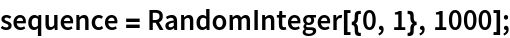Visualize the sequence:

 In:=Out=Apply a cumulative sums test:

 In:=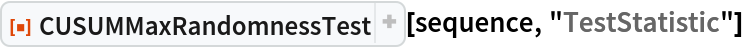Out=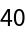In:=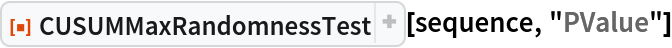Out=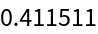### Applications (2)

Generate a sequence of integers from a random subsequence:

 In:=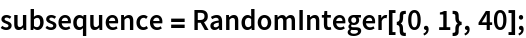In:=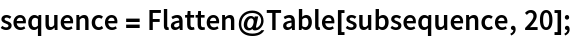Visualize the sequence:

 In:=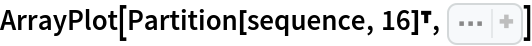Out=Apply a cumulative sums test. The small p-value indicates that the sequence is non-random and hence should be rejected:

 In:=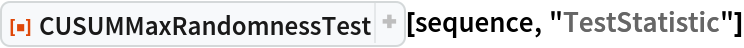Out=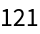In:=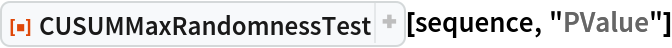Out=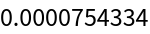Define and plot rule 30:

 In:=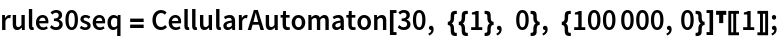In:=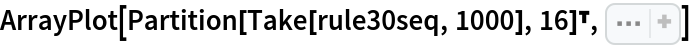Out=Test whether rule 30 is random:

 In:=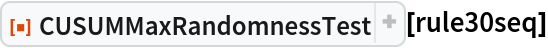Out=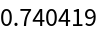### Possible Issues (1)

CUSUMMaxRandomnessTest requires sequences of length 100 or more:

 In:=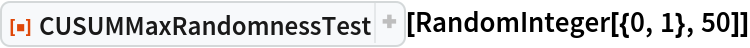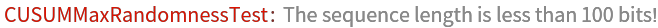### Neat Examples (1)

Visualize the sampling distribution of the test statistic:

 In:=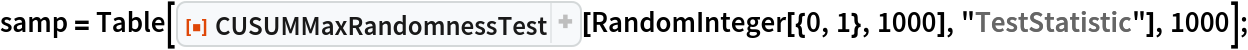In:=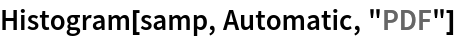Out=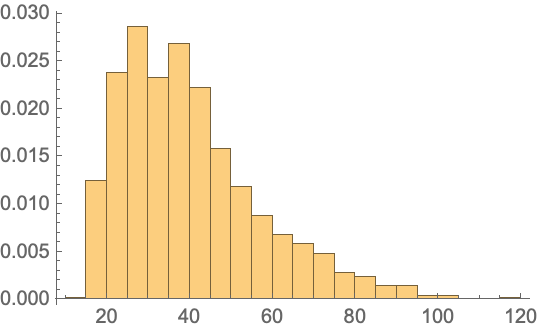Emmy Blumenthal

## Version History

• 1.0.0 – 08 July 2019